Contents

### Context

#### Duality in string theory

duality in string theory

general mechanisms

string-fivebrane duality

string-string dualities

M-theory

F-theory

string-QFT duality

QFT-QFT duality:

#### Quantum field theory

functorial quantum field theory

# Contents

## Idea

### General idea

The AdS-CFT correspondence at its heart is the observation (Witten 98, Section 2.4) that the classical action functionals for various fields coupled to Einstein gravity on anti de Sitter spacetime are, when expressed as functions of the asymptotic boundary-values of the fields, of the form of generating functions for correlators/n-point functions of a conformal field theory on that asymptotic boundary, in a large N limit.

This is traditionally interpreted as a concrete realization of a vague “holographic principle” according to which quantum gravity in bulk spacetimes is controlled, in one way or other, by “boundary field theories” on effective spacetime boundaries, such as event horizons. The original and main motivation for the holographic principle itself was the fact that the apparent black hole entropy in Einstein gravity scales with the area of the event horizon instead of the black hole’s bulk volume (which is not even well-defined), suggesting that gravity encodes or is encoded by some boundary field theory associated with horizons; an idea that, in turn, seems to find a concrete realization in open/closed string duality in the vicinity of, more generally, black branes. The original intuition about holographic black hole entropy has meanwhile found remarkably detailed reflection in (mathematically fairly rigorous) analysis of holographic entanglement entropy, specifically via holographic tensor networks, which turn out to embody key principles of the AdS/CFT correspondence in the guise of quantum information theory, with concrete applications such as to quantum error correcting codes.

The AdS/CFT correspondence itself crucially involves the exceptional isomorphism between the isometry group of anti de Sitter spacetime $AdS_{d+1}$ (the anti de Sitter group) and the conformal group of Minkowski spacetime of dimension $d$: the connected component of both is the special orthogonal group $SO(d,2)$. But the AdS/CFT correspondence is deeper and more subtle than this group theory underlying it, in particular in how it puts fields and states on the gravity side in correspondence with sources and correlators on the field theory side, respectively.

In extrapolation of these elementary computations, the AdS/CFT correspondence conjecturally extends to a more general identification of states of gravity (quantum gravity) on asymptotically anti de Sitter spacetimes of dimension $d+1$ with correlators/n-point functions of conformal field theories on the asymptotic boundary of dimension $d$ (Gubser-Klebanov-Polyakov 98 (12), Witten 98, (2.11)), such that perturbation theory on one side of the correspondence relates to non-perturbation on the other side.

While this works to some extent quite generally (see e.g. Natsuume 15 for review), allowing applications such as AdS/CFT in condensed matter physics and AdS/CFT in quantum chromodynamics, the tightest form of the correspondence relates the 1/N expansion of superconformal field theories (super Yang-Mills theories) on the asymptotic boundaries of near-horizon limits of $N$ coincident black M2-branes/D3-branes/M5-branes to corresponding sectors of the string theory/M-theory quantum gravity in the bulk spacetime away from the brane.

Before the proposal for the actual matching rule of ADS/CFT (Gubser-Klebanov-Polyakov 98 (12), Witten 98, (2.11)) it was by matching of BPS-states in these situations that the existence of an AdS/CFT correspondence was proposed in Maldacena 97a, Maldacena 97b; these articles are now widely regarded as the origin of the idea of the AdS/CFT correspondence.

A quick way to see that the supersymmetric-cases of AdS/CFT for near horizon geometries of M2-branes, D3-branes and M5-branes must be special is to observe that these are the only dimensions in which there are super anti de Sitter spacetime-enhancements of anti de Sitter spacetime, matching the classification of simple superconformal symmetries, see there:

$d$$N$superconformal super Lie algebraR-symmetryblack brane worldvolume
superconformal field theory
$\phantom{A}3\phantom{A}$$\phantom{A}2k+1\phantom{A}$$\phantom{A}B(k,2) \simeq$ osp$(2k+1 \vert 4)\phantom{A}$$\phantom{A}SO(2k+1)\phantom{A}$
$\phantom{A}3\phantom{A}$$\phantom{A}2k\phantom{A}$$\phantom{A}D(k,2)\simeq$ osp$(2k \vert 4)\phantom{A}$$\phantom{A}SO(2k)\phantom{A}$M2-brane
D=3 SYM
BLG model
ABJM model
$\phantom{A}4\phantom{A}$$\phantom{A}k+1\phantom{A}$$\phantom{A}A(3,k)\simeq \mathfrak{sl}(4 \vert k+1)\phantom{A}$$\phantom{A}U(k+1)\phantom{A}$D3-brane
D=4 N=4 SYM
D=4 N=2 SYM
D=4 N=1 SYM
$\phantom{A}5\phantom{A}$$\phantom{A}1\phantom{A}$$\phantom{A}F(4)\phantom{A}$$\phantom{A}SO(3)\phantom{A}$D4-brane
D=5 SYM
$\phantom{A}6\phantom{A}$$\phantom{A}k\phantom{A}$$\phantom{A}D(4,k) \simeq$ osp$(8 \vert 2k)\phantom{A}$$\phantom{A}Sp(k)\phantom{A}$M5-brane
D=6 N=(2,0) SCFT
D=6 N=(1,0) SCFT

(Shnider 88, also Nahm 78, see Minwalla 98, section 4.2)

It had already been observed in (Duff-Sutton 88, see Duff 98, Duff 99) that the field theory of small perturbation of a Green-Schwarz sigma-model for a fundamental brane stretched over the asymptotic boundary of the AdS near horizon geometry of its own black brane-incarnation is, after diffeomorphism gauge fixing, a conformal field theory. This was further developed in Claus-Kallosh-Proeyen 97, DGGGTT 98, Claus-Kallosh-Kumar-Townsend 98, Pasti-Sorokin-Tonin 99. See also at super p-brane – As part of the AdS-CFT correspondence

More recently, for the archetypical case of AdS/CFT relating N=4 D=4 super Yang-Mills theory to type IIB string theory on super anti de Sitter spacetime $AdS_5 \times S^5$, fine detailed checks of the correspondence have been performed (Beisert et al. 10, Escobedo 12), see the section Checks below.

Thus regarded as a duality in string theory, the AdS/CFT correspondence is an incarnation of open/closed string duality, reflecting the fact that the physics on D-branes has two equivalent descriptions:

1) as a Yang-Mills-gauge theory coming from open strings attached the brane

2) as a gravity theory coming from closed strings emitted/absorbed by the brane.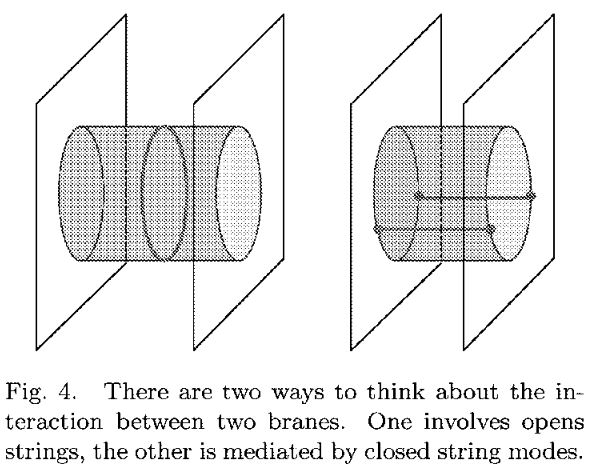graphics grabbed from Schomerus 07, Figure 4, see also e.g. Peschanskia 09, Figure 1

This gives a vivid intuitive picture of the mechanism underlying the correspondence: An excitation of the gauge field on the brane goes along with an excitation of the field of gravity around the brane, and either is faithfully reflected in the other; at least in the suitable limits.

### Small-$N$ corrections

The AdS/CFT correspondence has been widely discussed and is mostly understood by default only in the large $N$ limit and for large 't Hooft coupling, where the given gauge theory is dual to plain classical supergravity, which stands out as being particularly tractable and well-understood.

But it is expected (AGMOO99, p. 60) that the duality still applies in the opposite large 1/N limit, now involving on the gravity-side corrections

1. from perturbative string theory (for small 't Hooft coupling, there are some checks of such stringy corrections) and

2. from putative M-theory (for the full non-perturbative large 1/N limit, which remains largely unexplored):

$\,$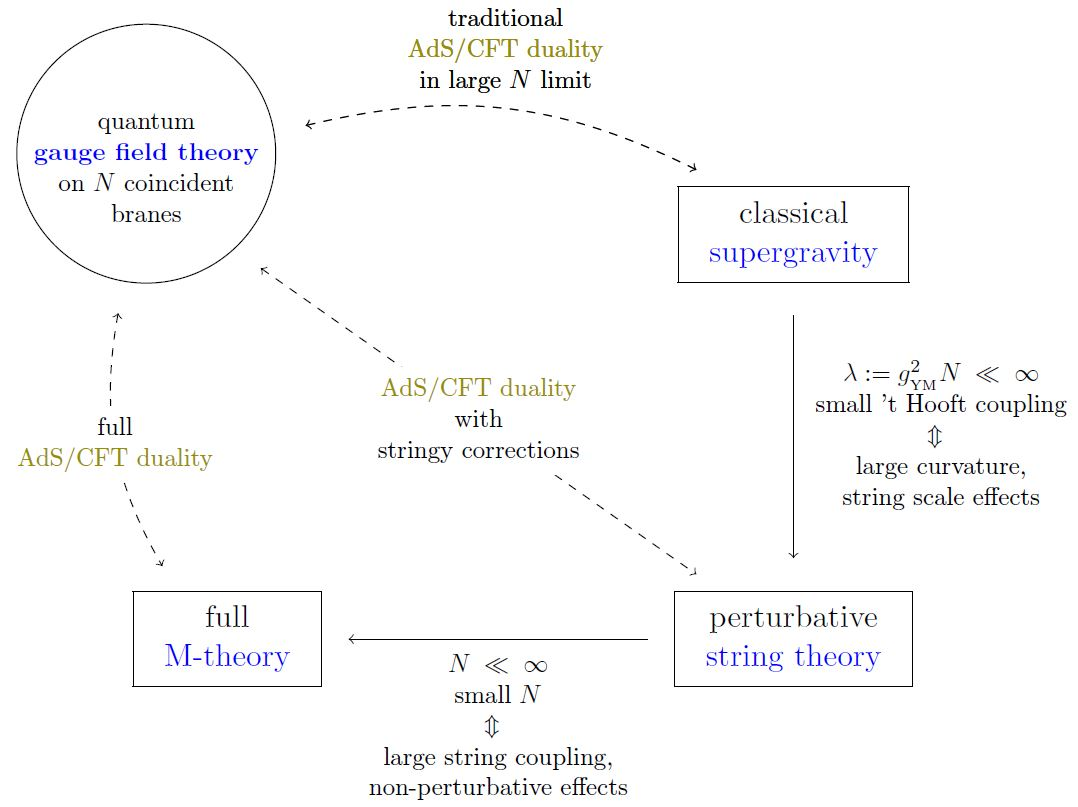(graphics from SS22)

Notice that for real-world applications such as to the confinement/mass gap-problem of quantum chromodynamics, the value of $N$ typically is indeed small (the number of colors in quantum chromodynamics is $N_c = 3$) so that the string theory/M-theory-corrections to the AdS/QCD correspondence are going to be crucial for the full discussion of these applications: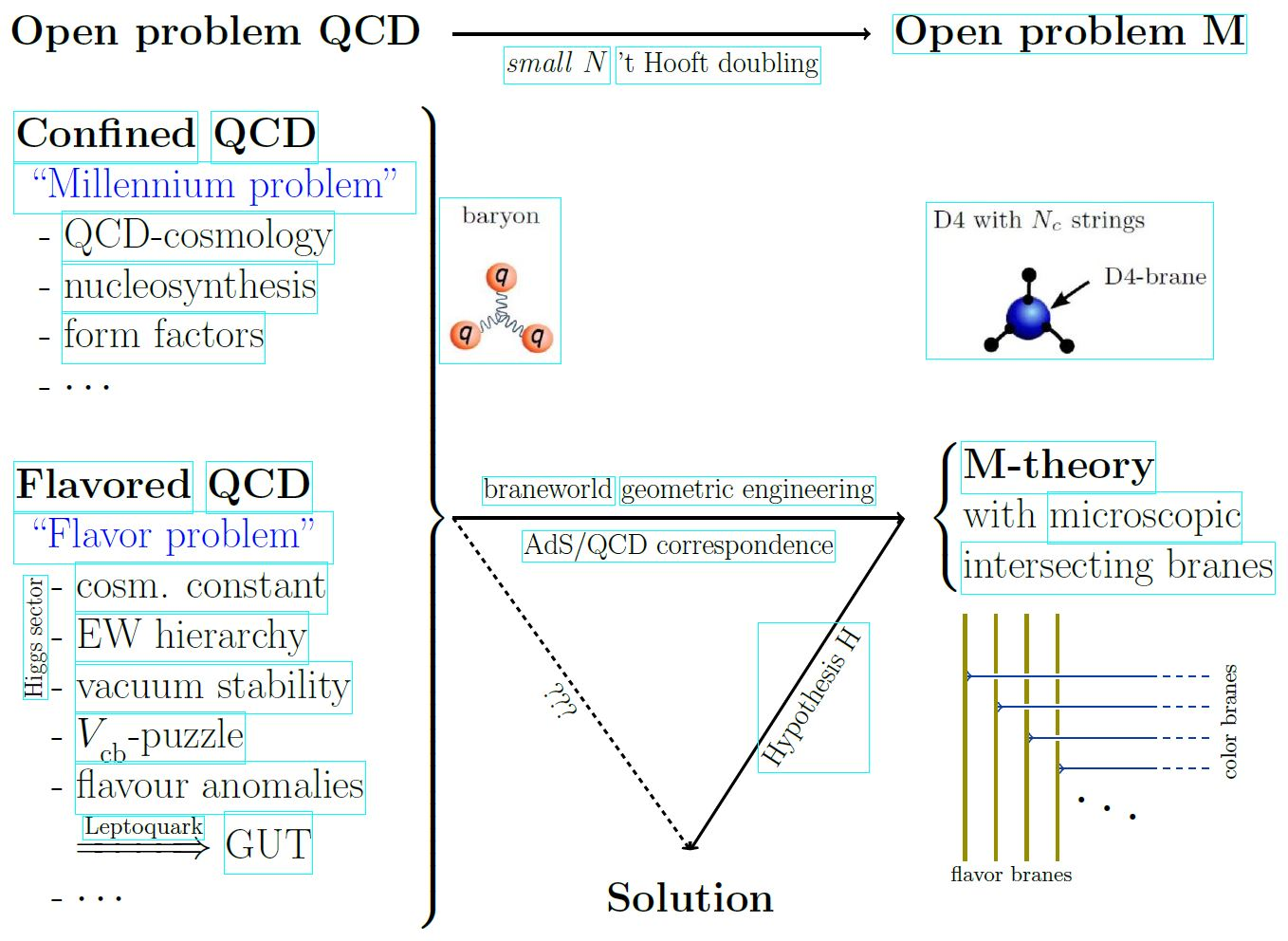In lack of a full formulation of M-theory (see M-theory – The open problem) approximate forms of the AdS/CFT correspondence away from the case of conformal invariance, supersymmetry, large N limit and/or exact anti de Sitter geometry are being argued to be of use for understanding quantum chromodynamics (for instance the quark-gluon plasma (Policastro-Son-Starinets 01, but most notably confined hadron-spectra – the AdS/QCD correspondence) and for various models in solid state physics (the AdS-CFT in condensed matter physics, see e.g. Hartnoll-Lucas-Sachdev 16).

More in detail, since the near horizon geometry of BPS black branes is conformal to the Cartesian product of anti de Sitter spaces with the unit $n$-sphere around the brane, the cosmology of intersecting D-brane models realizes the observable universe on the asymptotic boundary of an approximately anti de Sitter spacetime (see for instance Kaloper 04, Flachi-Minamitsuji 09). The basic structure is hence that of Randall-Sundrum models, but details differ, such as notably in warped throat geometries, see Uranga 05, section 18.

These warped throat models go back to Klebanov-Strassler 00 which discusses aspects of confinement in Yang-Mills theory on conincident ordinary and fractional D3-branes at the singularity of a warped conifold. See also Klebanov-Witten 98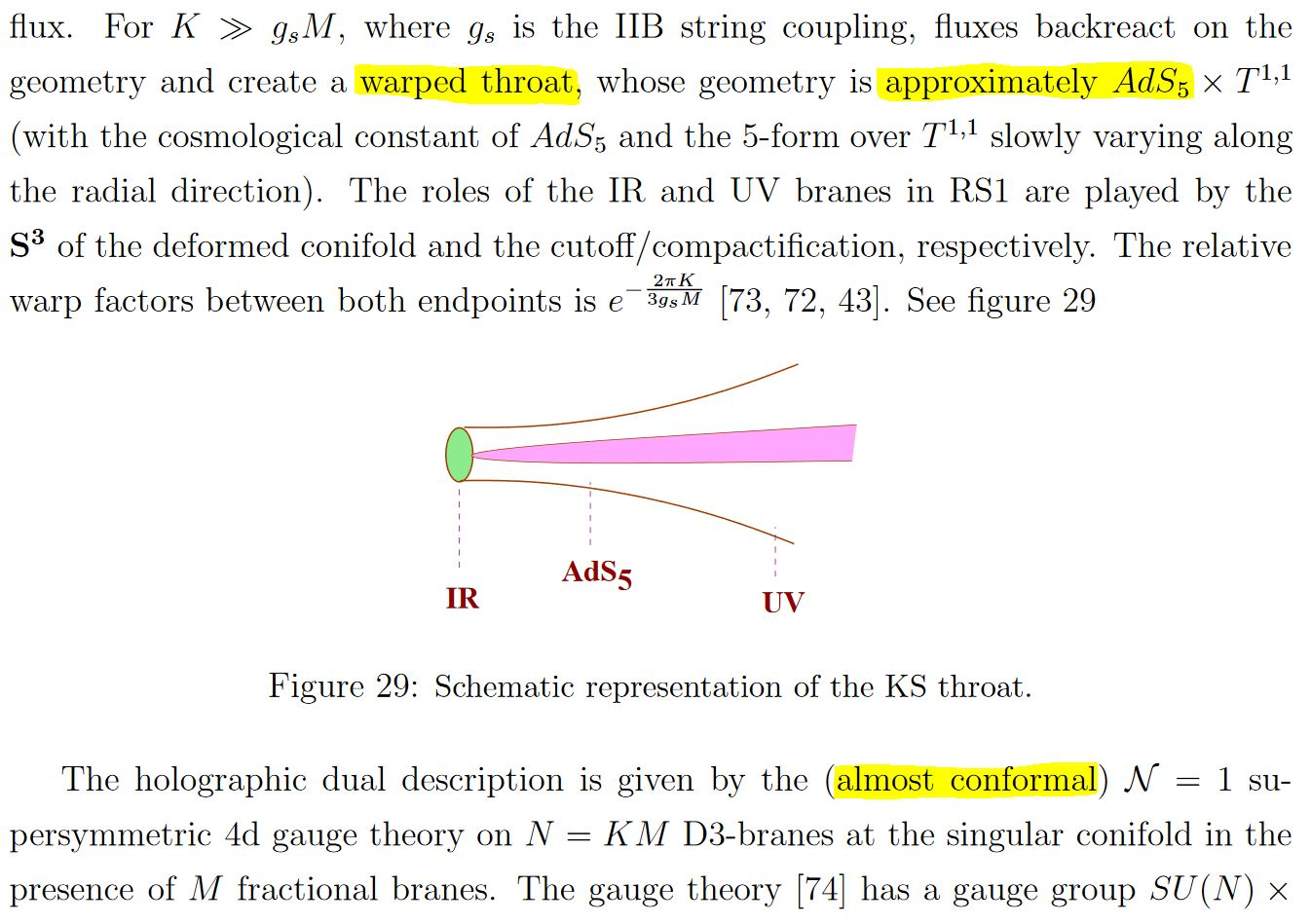snippet grabbed from Uranga 05, section 18

here: “RS”=Randall-Sundrum model; “KS”=Klebanov-Strassler 00

In particular this means that AdS-CFT duality applies in some approximation to intersecting D-brane models (e.g. Soda 10, GHMO 16), thus allowing to compute, to some approximation, non-perturbative effects in the Yang-Mills theory on the intersecting branes in terms of gravity on the ambient warped throat $\sim$ AdS (Klebanov-Strassler 00, section 6)

### Matching single trace observables to string excitation

The single trace operators/observables in conformal field theories such as super Yang-Mills theories play a special role in the AdS-CFT correspondence: They correspond to single string excitations on the AdS-supergravity side of the correspondence, where, curiously, the “string of characters/letters” in the argument of the trace gets literally mapped to a superstring in spacetime (see the references below).

From Polyakov 02, referring to gauge fields and their single trace operators as letter and words, respectively:

The picture which slowly arises from the above considerations is that of the space-time gradually disappearing in the regions of large curvature. The natural description in this case is provided by a gauge theory in which the basic objects are the texts formed from the gauge-invariant words. The theory provides us with the expectation values assigned to the various texts, words and sentences.

These expectation values can be calculated either from the gauge theory or from the strongly coupled 2d sigma model. The coupling in this model is proportional to the target space curvature. This target space can be interpreted as a usual continuous space-time only when the curvature is small. As we increase the coupling, this interpretation becomes more and more fuzzy and finally completely meaningless.

From Berenstein-Maldacena-Nastase 02, who write $Z$ for the elementary field observables (“letters”) $\mathbf{\Phi}$ above:

In summary, the “string of $Z$s” becomes the physical string and that each $Z$ carries one unit of $J$ which is one unit of $p_+$. Locality along the worldsheet of the string comes from the fact that planar diagrams allow only contractions of neighboring operators. So the Yang Mills theory gives a string bit model where each bit is a $Z$ operator.

On the CFT side these BMN operators of fixed length (of “letters”) are usefully identified as spin chains which, with the dilatation operator regarded as their Hamiltonian, are integrable systems (Minahan-Zarembo 02, Beisert-Staudacher 03).

This integrability allows a detailed matching between

under AdS/CFT duality (Beisert-Frolov-Staudacher-Tseytlin 03, …). For review see BBGK 04, Beisert et al. 10.

(…)

## Checks

At the heart of the duality is the observation that the classical action functionals for various fields coupled to Einstein gravity on anti de Sitter spacetime are, when expressed as functions of the asymptotic boundary values of the fields, equal to the generating functions for the correlators/n-point functions of a conformal field theory on that asymptotic boundary.

These computations were laid out in Witten 98, section 2.4 “Some sample computation”. These follow from elementary manipulation in differential geometry (involving neither supersymmetry nor string theory). A good exposition is in Hartnoll-Lucas-Sachdev 16, Section 1.6

For the more ambitious matching of the spectrum of the dilatation operator of N=4 D=4 super Yang-Mills theory to the corresponding spectrum of the Green-Schwarz superstring on the super anti de Sitter spacetime $AdS_5 \times S^5$ detailed checks are summarized in Beisert et al. 10, Escobedo 12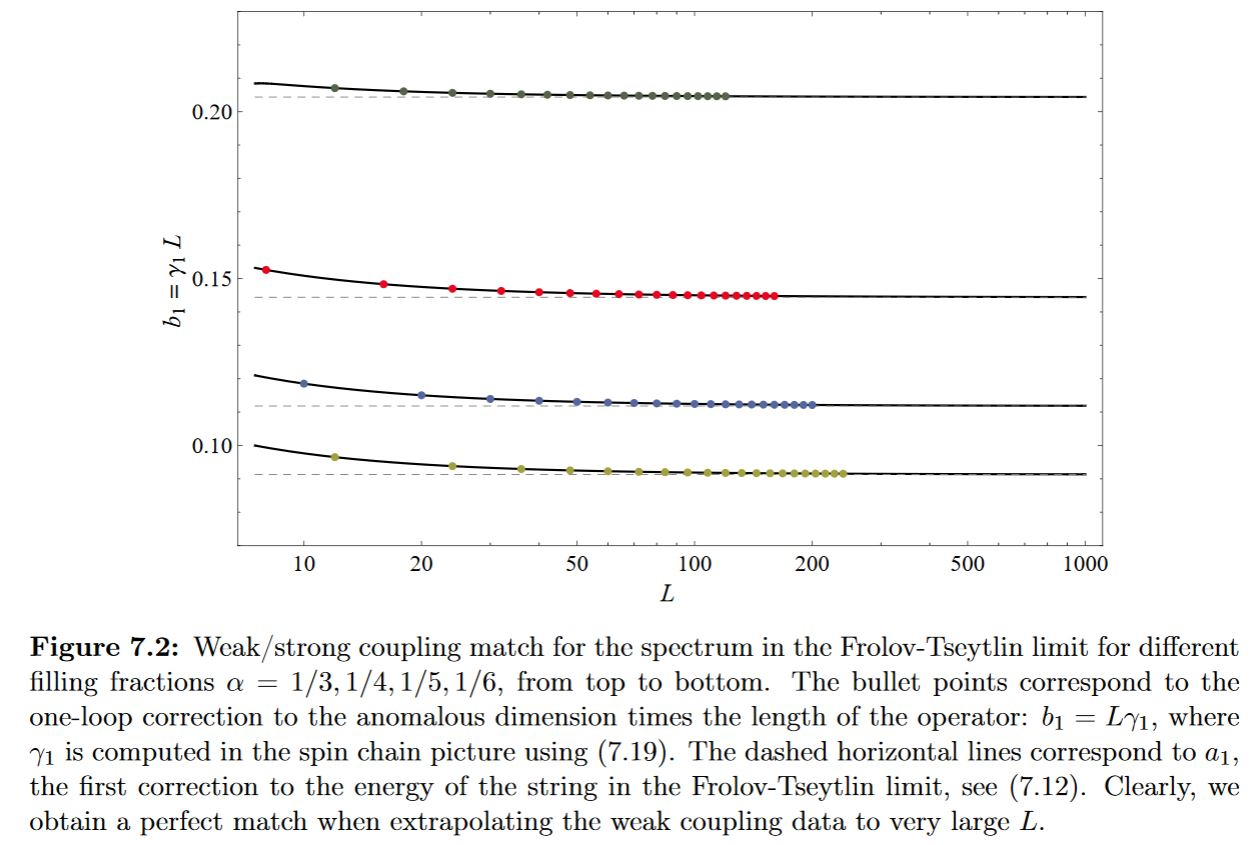graphics grabbed from Escobedo 12

Comparison to string scattering amplitudes beyond the planar SCFT limit: ABP 18.

Numerical checks using lattice gauge theory are reviewed in Joseph 15.

Exact duality checks pertaining to the full stringy regime for $AdS_3/CFT_2$: Eberhardt-Gaberdiel 19a, Eberhardt-Gaberdiel 19b, Eberhardt-Gaberdiel-Gopakumar 19. For more see the references there.

## Examples

The solutions to supergravity that preserve the maximum of 32 supersymmetries are (e.g. HEGKS 08 (1.1))

as well as their Minkowski spacetime and plane wave limits. These are the main KK-compactifications for the following examples-

### $AdS_5 / CFT_4$ – Horizon limit of D3-branes

type II string theory on 5d anti de Sitter spacetime (times a 5-sphere) is dual to N=4 D=4 super Yang-Mills theory on the worldvolume of a D3-brane at the asymptotic boundary

### $AdS_7 / CFT_6$ – Horizon limit of M5-branes

We list some of the conjectured statements and their evidence concerning the case of $AdS_7/CFT_6$-duality.

The hypothesis (Maldacena 97, section 3.1) (see (Aharony-Gubser-Maldacena-Ooguri-Oz 99, section 6.1.1) for a review) is that

is holographically related to

In

effectively this relation was already used to computed the 5-brane partition function in the abelian case from the states of abelian 7d Chern-Simons theory. (The quadratic refinement of the supergravity C-field necessary to make this come out right is what led to Hopkins-Singer 02 and hence to the further mathematical development of differential cohomology and its application in physics.)

In (Witten 98, section 4) this construction is argued for from within the framework of AdS/CFT, explicitly identifying the 7d Chern-Simons theory here with the compactification of the 11-dimensional Chern-Simons term of the supergravity C-field in 11-dimensional supergravity, which locally is

\begin{aligned} S_{11d SUGRA, CS}(C_3) &= \int_{AdS_7} \int_{S^4} C_3 \wedge G_4 \wedge G_4 \\ & = N \, \int_{AdS_7} C_3 \wedge G_4 \end{aligned} \,.

But in fact the quantum anomaly cancellation (GS-type mechanism) for 11d sugra introduces a quantum correction to this Chern-Simons term (DLM, equation (3.14)), making it locally become

\begin{aligned} S(\omega,C_3) &= \int_{AdS_7} \int_{S^4} C_3 \wedge G_4 \wedge (G_4 + I_8(\omega)) \\ & = N \, \int_{AdS_7} \left( C_3 \wedge G_4 + \frac{1}{48} CS_{p_2}(\omega) - \frac{1}{12} CS_{\frac{1}{2}p_1}(\omega) \wedge tr(F_\omega \wedge \omega) \right) \end{aligned} \,,

where now $\omega$ is the local 1-form representative of a spin connection and where $CS_{p_2}$ is a Chern-Simons form for the second Pontryagin class and $CS_{\frac{1}{2}p_1}$ for the first.

That therefore not an abelian, but this nonabelian higher dimensional Chern-Simons theory should be dual to the nonabelian 6d (2,0)-superconformal QFT was maybe first said explicitly in (LuWang 2010).

Its gauge field is hence locally and ignoring the flux quantization subtleties a pair consisting of the abelian 3-form field $C$ and a Spin group $Spin(6,1)$-valued connection (see supergravity C-field for global descriptions of such pairs). Or maybe rather $Spin(6,2)$ to account for the constraint that the configurations are to be asymptotic anti de Sitter spacetimes (in analogy to the well-understood situation in 3d quantum gravity, see there for more details).

Indeed, in (SezginSundell 2002, section 7) more detailed arguments are given that the 7-dimensional dual to the free 6d theory is a higher spin gauge theory for a higher spin gauge group extending the (super) conformal group $SO(6,2)$.

A non-perturbative description of this nonabelian 7d Chern-Simons theory as a local prequantum field theory (hence defined non-perturbatively on the global moduli stack of fields (twisted differential string structures, in fact)) was discussed in (FSS 12a, FSS 12b).

General discussion of boundary local prequantum field theories relating higher Chern-Simons-type and higher WZW-type theories is in (dcct 13, section 3.9.14). Specifically, a characterization along these lines of the Green-Schwarz action functional of the M5-brane as a holographic higher WZW-type boundary theory of a 7d Chern-Simons theory is found in (FSS 13).

Analogous discussion of the 6d theory as a higher WZW analog of a 7d Chern-Simons theory phrased in terms of extended quantum field theory is (Freed 12).

### $AdS_4 / CFT_3$ –Horizon limit of M2-branes

11d supergravity/M-theory on the asymptotic $AdS_4$

spacetime of an M2-brane.

### $AdS_3 / CFT_2$ – Horizon limit of D$p$-D($p+k$) brane bound states

(for more see at AdS3-CFT2 and CS-WZW correspondence)

(Dibitetto-Petri 17, …)

(Dibitetto-Petri 18, …)

### $AdS_2 / CFT_1$

see at nearly AdS2/CFT1

### Non-conformal duals

#### QCD models

While all of the above horizon limits product super Yang-Mills theory, one can consider certain limits of these in which they look like plain QCD, at least in certain sectors. This leads to a discussion of holographic description of QCD properties that are actually experimentally observed.

### Further gauge theories induced by compactification and twisting

gauge theory induced via AdS-CFT correspondence

M-theory perspective via AdS7-CFT6F-theory perspective
11d supergravity/M-theory
$\;\;\;\;\downarrow$ Kaluza-Klein compactification on $S^4$compactificationon elliptic fibration followed by T-duality
7-dimensional supergravity
$\;\;\;\;\downarrow$ topological sector
7-dimensional Chern-Simons theory
$\;\;\;\;\downarrow$ AdS7-CFT6 holographic duality
6d (2,0)-superconformal QFT on the M5-brane with conformal invarianceM5-brane worldvolume theory
$\;\;\;\; \downarrow$ KK-compactification on Riemann surfacedouble dimensional reduction on M-theory/F-theory elliptic fibration
N=2 D=4 super Yang-Mills theory with Montonen-Olive S-duality invariance; AGT correspondenceD3-brane worldvolume theory with type IIB S-duality
$\;\;\;\;\; \downarrow$ topological twist
topologically twisted N=2 D=4 super Yang-Mills theory
$\;\;\;\; \downarrow$ KK-compactification on Riemann surface
A-model on $Bun_G$, Donaldson theory

$\,$

gauge theory induced via AdS5-CFT4
type II string theory
$\;\;\;\;\downarrow$ Kaluza-Klein compactification on $S^5$
$\;\;\;\; \downarrow$ topological sector
5-dimensional Chern-Simons theory
$\;\;\;\;\downarrow$ AdS5-CFT4 holographic duality
N=4 D=4 super Yang-Mills theory
$\;\;\;\;\; \downarrow$ topological twist
topologically twisted N=4 D=4 super Yang-Mills theory
$\;\;\;\; \downarrow$ KK-compactification on Riemann surface
A-model on $Bun_G$ and B-model on $Loc_G$, geometric Langlands correspondence

## Formalizations

The full formalization of AdS/CFT is still very much out of reach, but maybe mostly for lack of trying.

But see Anderson 04.

One proposal for a formalization of a toy version in the context of AQFT is Rehren duality. However, it does not seem that this actually formalizes AdS-CFT, but something else.

Table of branes appearing in supergravity/string theory (for classification see at brane scan).

branein supergravitycharged under gauge fieldhas worldvolume theory
black branesupergravityhigher gauge fieldSCFT
D-branetype IIRR-fieldsuper Yang-Mills theory
$(D = 2n)$type IIA$\,$$\,$
D(-2)-brane$\,$$\,$
D0-brane$\,$$\,$BFSS matrix model
D2-brane$\,$$\,$$\,$
D4-brane$\,$$\,$D=5 super Yang-Mills theory with Khovanov homology observables
D6-brane$\,$$\,$D=7 super Yang-Mills theory
D8-brane$\,$$\,$
$(D = 2n+1)$type IIB$\,$$\,$
D(-1)-brane$\,$$\,$$\,$
D1-brane$\,$$\,$2d CFT with BH entropy
D3-brane$\,$$\,$N=4 D=4 super Yang-Mills theory
D5-brane$\,$$\,$$\,$
D7-brane$\,$$\,$$\,$
D9-brane$\,$$\,$$\,$
(p,q)-string$\,$$\,$$\,$
(D25-brane)(bosonic string theory)
NS-branetype I, II, heteroticcircle n-connection$\,$
string$\,$B2-field2d SCFT
NS5-brane$\,$B6-fieldlittle string theory
D-brane for topological string$\,$
A-brane$\,$
B-brane$\,$
M-brane11D SuGra/M-theorycircle n-connection$\,$
M2-brane$\,$C3-fieldABJM theory, BLG model
M5-brane$\,$C6-field6d (2,0)-superconformal QFT
M9-brane/O9-planeheterotic string theory
M-wave
topological M2-branetopological M-theoryC3-field on G2-manifold
topological M5-brane$\,$C6-field on G2-manifold
S-brane
SM2-brane,
membrane instanton
M5-brane instanton
D3-brane instanton
solitons on M5-brane6d (2,0)-superconformal QFT
self-dual stringself-dual B-field
3-brane in 6d

### Original articles

The rough conjecture originates in:

• Juan Maldacena, The Large $N$ limit of superconformal field theories and supergravity, Adv. Theor. Math. Phys. 2:231, 1998 (hep-th/9711200)

• Juan Maldacena, Wilson loops in Large $N$ field theories, Phys. Rev. Lett. 80 (1998) 4859 (hep-th/9803002)

The actual rule for matching bulk states to generating functions for boundary correlators/n-point functions is due to

Discussion of how Green-Schwarz action functionals for super $p$-branes in AdS target spaces induce, after diffeomorphism gauge fixing, superconformal field theory on the worldvolumes (see singleton representation) goes back to

• Mike Duff, C. Sutton, The Membrane at the End of the Universe, New Sci. 118 (1988) 67-71 (spire:268230)

and was further developed in

Review is in

The resulting super-conformal brane scan:

Sketch of a derivation of AdS/CFT:

Further references include:

• Oscar Varela, Super-Chern-Simons spectra from Exceptional Field Theory (arXiv:2010.09743)

### Introductions and surveys

Surveys and introductions include

Review of Yangian symmetry:

### Lattice gauge theory computations

Review of lattice gauge theory-numerics for the AdS-CFT correspondence:

• Anosh Joseph, Review of Lattice Supersymmetry and Gauge-Gravity Duality (arXiv:1509.01440)

Using the KK-compactification of D=4 N=4 super Yang-Mills theory to the BMN matrix model for lattice gauge theory-computations in D=4 N=4 SYM and for numerical checks of the AdS-CFT correspondence:

• Masazumi Honda, Goro Ishiki, Sang-Woo Kim, Jun Nishimura, Asato Tsuchiya, Direct test of the AdS/CFT correspondence by Monte Carlo studies of N=4 super Yang-Mills theory, JHEP 1311 (2013) 200 (arXiv:1308.3525)

### On single trace operators

The correspondence of single trace operators to superstring excitations under the AdS-CFT correspondence originates with these articles:

The identification of the relevant single trace operators with integrable spin chains is due to

which led to more detailed matching of single trace operators to rotating string excitations in

Review includes

### SYK-model in $AdS_2/CFT_1$

Discussion of the SYK-model as the AdS/CFT dual of JT-gravity in nearly AdS2/CFT1 and AdS-CFT in condensed matter physics:

Original articles:

Review:

Relation to black holes in terms of Majorana dimer states:

• Ioanna Kourkoulou, Juan Maldacena, Pure states in the SYK model and nearly-$AdS_2$ gravity (arXiv:1707.02325)

Relation to black holes in string theory and random matrix theory:

• Yuri D. Lensky, Xiao-Liang Qi, Pengfei Zhang, Size of bulk fermions in the SYK model (arXiv:2002.01961)

• Xiao-Liang Qi, Pengfei Zhang, The Coupled SYK model at Finite Temperature (arXiv:2003.03916)

• Akash Goel, Herman Verlinde, Towards a String Dual of SYK (arXiv:2103.03187)

• Tarek Anous, Felix M. Haehl, The quantum $p$-spin glass model: A user manual for holographers (arXiv:2106.03838)

Discussion of small N corrections via a lattice QFT-Ansatz on the AdS side:

• Richard C. Brower, Cameron V. Cogburn, A. Liam Fitzpatrick, Dean Howarth, Chung-I Tan, Lattice Setup for Quantum Field Theory in $AdS_2$ (arXiv:1912.07606)

• Gregory J. Galloway, Melanie Graf, Eric Ling, A conformal infinity approach to asymptotically $AdS_2 \times S^{n-1}$ spacetimes (arXiv:2003.00093)

### BFSS matrix model in $AdS_2/CFT_1$

On AdS2/CFT1 with the BFSS matrix model on the CFT side and black hole-like solutions in type IIA supergravity on the AdS side:

and on its analog of holographic entanglement entropy:

• Takeshi Morita, Hiroki Yoshida, A Critical Dimension in One-dimensional Large-N Reduced Models (arXiv:2001.02109)

### On $AdS_3 / CFT_2$

(For more see the references at AdS3/CFT2.)

An exact correspondence of the symmetric orbifold CFT of Liouville theory with a string theory on $AdS_3$ is claimed in:

based on

On black$\;$D6-D8-brane bound states in massive type IIA string theory, with defect D2-D4-brane bound states inside them realizing AdS3-CFT2 “inside” AdS7-CFT6:

On black$\;$D4-D8-brane bound states in massive type IIA string theory, with defect D2-D6-brane bound states inside them realizing AdS3-CFT2 “inside” AdS7-CFT6:

### On $AdS_5 / CFT_4$

Computing dual string scattering amplitudes by AdS/CFT beyond the planar limit:

### On $AdS_7 / CFT_6$

We list references specific to $AdS_7/CFT_6$.

In

it is argued that the conformal blocks of the 6d (2,0)-superconformal QFT are entirely controled just by the effective 7d Chern-Simons theory inside 11-dimensional supergravity, but only the abelian piece is discussed explicitly.

The fact that this Chern-Simons term is in fact a nonabelian higher dimensional Chern-Simons theory in $d = 7$, due the quantum anomaly cancellation, is clear from the original source, equation (3.14) of

but seems not to be noted explicitly in the context of $AdS_7/CFT_6$ before the references

• H. Lü, Yi Pang, Seven-Dimensional Gravity with Topological Terms Phys.Rev.D81:085016 (2010) (arXiv:1001.0042)

• H. Lu, Zhao-Long Wang, On M-Theory Embedding of Topologically Massive Gravity Int.J.Mod.Phys.D19:1197 (2010) (arXiv:1001.2349)

More on the relation between the M5-brane and supergravity on $AdS_7 \times S^4$ and arguments for the $SO(5)$ R-symmetry group on the 6d theory from the 7d theory are given in

• M. Nishimura, Y. Tanii, Local Symmetries in the $AdS_7/CFT_6$ Correspondence, Mod. Phys. Lett. A14 (1999) 2709-2720 (arXiv:hep-th/9910192)

Discussion of the $CFT_6$ in $AdS_7/CFT_6$ via conformal bootstrap:

In

arguments are given that the 7d theory is a higher spin gauge theory extension of $SO(6,2)$.

### Generalization beyond exact AdS / exact CFT

Discussion for cosmology of intersecting D-brane models (ambient $\sim$ anti de Sitter spacetimes with the $\sim$ conformal intersecting branes at the asymptotic boundary) includes the following (see also at Randall-Sundrum model):

• Igor Klebanov, Matthew Strassler, Supergravity and a Confining Gauge Theory: Duality Cascades and $\chi^{SB}$-Resolution of Naked Singularities, JHEP 0008:052, 2000 (arXiv:hep-th/0007191)

• Igor Klebanov, Edward Witten, Superconformal Field Theory on Threebranes at a Calabi-Yau Singularity, Nucl.Phys.B536:199-218, 1998 (arXiv:hep-th/9807080)

• Nemanja Kaloper, Origami World, JHEP 0405 (2004) 061 (arXiv:hep-th/0403208)

• Angel Uranga, section 18 of TASI lectures on String Compactification, Model Building, and Fluxes, 2005 (pdf)

• Antonino Flachi, Masato Minamitsuji, Field localization on a brane intersection in anti-de Sitter spacetime, Phys.Rev.D79:104021, 2009 (arXiv:0903.0133)

• Jiro Soda, AdS/CFT on the brane, Lect.Notes Phys.828:235-270, 2011 (arXiv:1001.1011)

• Shunsuke Teraguchi, around slide 21 String theory and its relation to particle physics, 2007 (pdf)

• Gianluca Grignani, Troels Harmark, Andrea Marini, Marta Orselli, The Born-Infeld/Gravity Correspondence, Phys. Rev. D 94, 066009 (2016) (arXiv:1602.01640)

### pp-Waves as Penrose limits of $AdS_p \times S^q$ spacetimes

Discussion of pp-wave spacetimes as Penrose limits (Inönü-Wigner contractions) of AdSp x S^q spacetimes and of the corresponding limit of AdS-CFT duality:

Review:

• Michael Gutperle, Nicholas Klein, A Penrose limit for type IIB $AdS_6$ solutions (arXiv:2105.10824)

### Applications to physics

#### To gravity

Discussion of event horizons of black holes in terms of AdS/CFT (the “firewall problem”) is in

• Kyriakos Papadodimas, Suvrat Raju, An Infalling Observer in AdS/CFT (arXiv:1211.6767)

To black hole interiors:

The SYK model gives us a glimpse into the interior of an extremal black hole…That’s the feature of SYK that I find most interesting…It is a feature this model has, that I think no other model has

To symmetries in gravity:

#### To the quark-gluon plasma

Applications of AdS-CFT to the quark-gluon plasma of QCD:

Expositions and reviews include

Holographic discussion of the shear viscosity of the quark-gluon plasema goes back to

Other original articles include:

• Hovhannes R. Grigoryan, Paul M. Hohler, Mikhail A. Stephanov, Towards the Gravity Dual of Quarkonium in the Strongly Coupled QCD Plasma (arXiv:1003.1138)

• Brett McInnes, Holography of the Quark Matter Triple Point (arXiv:0910.4456)

#### To particle physics

For more see at AdS/QCD correspondence.

#### To fluid dynamics

Application to fluid dynamics – see also at fluid/gravity correspondence:

#### To condensed matter physics

Textbook account

Further reviews include the following:

### Applications in mathematics

#### To the volume conjecture

Suggestion that the statement of the volume conjecture is really AdS-CFT duality combined with the 3d-3d correspondence for M5-branes wrapped on hyperbolic 3-manifolds:

• Dongmin Gang, Nakwoo Kim, Sangmin Lee, Section 3.2_Holography of 3d-3d correspondence at Large $N$, JHEP04(2015) 091 (arXiv:1409.6206)

• Dongmin Gang, Nakwoo Kim, around (21) of: Large $N$ twisted partition functions in 3d-3d correspondence and Holography, Phys. Rev. D 99, 021901 (2019) (arXiv:1808.02797)

#### To deep learning in neural networks

On the deep learning algorithm on neural networks as analogous to the AdS/CFT correspondence:

• Yi-Zhuang You, Zhao Yang, Xiao-Liang Qi, Machine Learning Spatial Geometry from Entanglement Features, Phys. Rev. B 97, 045153 (2018) (arxiv:1709.01223)

• W. C. Gan and F. W. Shu, Holography as deep learning, Int. J. Mod. Phys. D 26, no. 12, 1743020 (2017) (arXiv:1705.05750)

• J. W. Lee, Quantum fields as deep learning (arXiv:1708.07408)

• Koji Hashimoto, Sotaro Sugishita, Akinori Tanaka, Akio Tomiya, Deep Learning and AdS/CFT, Phys. Rev. D 98, 046019 (2018) (arxiv:1802.08313)